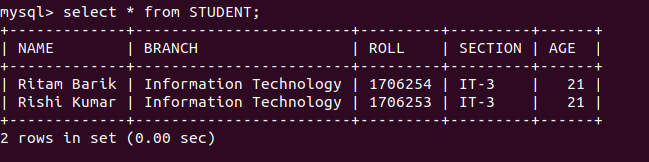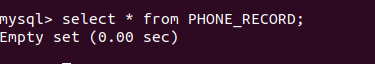GFG App
Open AppBrowser
Continue

Python Database API ( Application Program Interface ) is the Database interface for the standard Python. This standard is adhered to by most Python Database interfaces. There are various Database servers supported by Python Databases such as MySQL, GadFly, PostgreSQL, Microsoft SQL Server 2000, Informix, Interbase, Oracle, Sybase, etc. To connect with MySQL database server from Python, we need to import the mysql.connector interface. Below is a program to connect with MySQL database geeks.

## Python

 `# importing required library ` `import` `mysql.connector`   `# connecting to the database ` `dataBase ``=` `mysql.connector.connect(` `                     ``host ``=` `"localhost",` `                     ``user ``=` `"user",` `                     ``passwd ``=` `"pswrd",` `                     ``database ``=` `"geeks" ) `   `# preparing a cursor object ` `cursorObject ``=` `dataBase.cursor() `     `# disconnecting from server` `dataBase.close() `

The above program illustrates the connection with the MySQL database geeks in which host-name is localhost, the username is user and password is pswrd.

### Deleting query from tables

After connecting with the database in MySQL we can create tables in it and can manipulate them. Syntax Statement:

`DELETE FROM TABLE_NAME WHERE ATTRIBUTE_NAME = ATTRIBUTE_VALUE`

Example 1: Below is a program to delete a query from the table in the database.

## Python

 `# importing required library ` `import` `mysql.connector `   `# connecting to the database ` `dataBase ``=` `mysql.connector.connect(` `                     ``host ``=` `"localhost",` `                     ``user ``=` `"user",` `                     ``passwd ``=` `"pswrd",` `                     ``database ``=` `"geeks" )  `   `# preparing a cursor object ` `cursorObject ``=` `dataBase.cursor() `   `# creating table  ` `studentRecord ``=` `"""CREATE TABLE STUDENT ( ` `                   ``NAME  VARCHAR(``20``) NOT NULL, ` `                   ``BRANCH VARCHAR(``50``), ` `                   ``ROLL ``INT` `NOT NULL,` `                   ``SECTION VARCHAR(``5``), ` `                   ``AGE ``INT` `                   ``)"""`   `# table created` `cursorObject.execute(studentRecord)  `   `# inserting data into the table` `query ``=` `"INSERT INTO STUDENT (NAME, BRANCH, ROLL, SECTION, AGE) VALUES (``%` `s, ``%` `s)"`   `attrValues ``=` `("Rituraj Saha", "Information Technology", "``1706256``", "IT``-``3``", "``20``")` `cursorObject.execute(query, attrValues)`   `attrValues ``=` `("Ritam Barik", "Information Technology", "``1706254``", "IT``-``3``", "``21``")` `cursorObject.execute(query, attrValues)`   `attrValues ``=` `("Rishi Kumar", "Information Technology", "``1706253``", "IT``-``3``", "``21``")` `cursorObject.execute(query, attrValues)`   `# deleting query` `query ``=` `"DELETE FROM STUDENT WHERE ROLL ``=` `1706256``"` `cursorObject.execute(query, attrValues)`   `dataBase.commit()`   `# disconnecting from server` `dataBase.close()`

Output:In the above program, a table named STUDENT is created having attributes NAME, BRANCH, ROLL, SECTION and AGE. Multiple data is inserted into the STUDENT table and then a single query is deleted from the table having the ROLL attribute value 1706256. Example 2: Let us look at another example for queries in a table.

## Python

 `# importing required library ` `import` `mysql.connector`   `# connecting to the database ` `dataBase ``=` `mysql.connector.connect(` `                     ``host ``=` `"localhost",` `                     ``user ``=` `"user",` `                     ``passwd ``=` `"pswrd",` `                     ``database ``=` `"geeks" ) `   `# preparing a cursor object ` `cursorObject ``=` `dataBase.cursor() `   `# drop table if it already exists ` `cursorObject.execute("DROP TABLE IF EXISTS PHONE_RECORD")`   `# creating table  ` `phoneRecord ``=` `"""CREATE TABLE PHONE_RECORD ( ` `                   ``NAME  VARCHAR(``20``) NOT NULL, ` `                   ``PHONE VARCHAR(``10``) NOT NULL` `                   ``)"""`   `# table created` `cursorObject.execute(phoneRecord)  `   `# inserting data into the table` `query ``=` `"INSERT INTO PHONE_RECORD (NAME, PHONE) VALUES (``%` `s, ``%` `s)"` `attrValues ``=` `("Rituraj Saha", "``9163089075``")` `cursorObject.execute(query, attrValues)`   `# deleting query` `query ``=` `"DELETE FROM STUDENT WHERE NAME ``=` `'Rituraj Saha'``"` `cursorObject.execute(query)`   `dataBase.commit()`   `# disconnecting from server` `dataBase.close()`

Output:In the above program, another table is created in the geeks database named PHONE_RECORD having attribute NAME and PHONE. Only one column is inserted into the table and then it is deleted using the DELETE statement.

My Personal Notes arrow_drop_up